Next: 3.3 Normalization/weighting of the Up: 3. Problems with the Previous: 3.1 Bad conditioning

## 3.2 Nonlinearity

If the matrices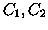and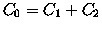are positive-definite it is possible to compute a linear proposed identification; but is the linear formalism a reasonable approximation of the full nonlinear problem?

This difficulty is not a separate one from the bad conditioning one: if the conditioning number ofis very large, there is a very large eigenvalueand a very small one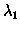. Then the inverse matrixmust have a small eigenvalueand a large eigenvalue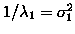, and a corresponding eigenspace along which the RMS uncertainty is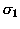; this is also the length of the longest semi-axis of the confidence ellipsoid defined by the inequality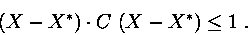When the longest axis of the confidence ellipsoid is very long at the central epoch the linear approximation is not adequate. However, even if it is adequate at the central epoch, the along track uncertainty grows with time, and when the confidence region spans several degrees in mean longitude the linear approximation may fail.

The problem of nonlinearity does not have an easy solution, applicable to the orbit identification problem; brute force'' (that is computationally intensive) solutions can be applied to analyze a couple of orbits for an already suspected identification, but cannot be used to propose identifications, that is to select among hundred of millions couples of orbits.

One possibility is to use a reduced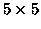covariance matrix, as in our tests in Section 4. Another solution is to discard the couples of orbitsandsuch that the difference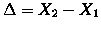is too large, by using a simple metric, even when the linear identification algorithm would indicate the couple as a possible identification. This solution is quite unsatisfactory, because one of the goals of the algorithm we are proposing is to allow identification of couples even with large; the cases with smallcould be found, and indeed are at present found, with much simpler algorithms, such as the one used in [Sansaturio et al. 1996].

An additional problem of nonlinearity is introduced if a singular set of elements is used. For example, if the least squares fit is performed with the state vectorexpressed in the usual keplerian elements, the linear approximation breaks down at the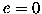and/or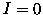singularities of the keplerian elements. If the confidence region containsand/or, then the linear approximation necessarily fails within the confidence region, even when the latter is small. This problem is easily solved by using nonsingular elements such as the equinoctial ones, for which the covariance propagation is more regular; see Paper I, Section 4.1.Next: 3.3 Normalization/weighting of the Up: 3. Problems with the Previous: 3.1 Bad conditioning

Maria Eugenia Sansaturio
1999-05-20